Next Article in Journal
Remuneration Committee, Board Independence and Top Executive Compensation
Previous Article in Journal
Revisiting the Performance of MACD and RSI Oscillators
Open AccessArticle

# Validation of the Merton Distance to the Default Model under Ambiguity

Department of Quantitative Finance, National Tsing Hua University, Hsinchu 30013, Taiwan
*
Author to whom correspondence should be addressed.
J. Risk Financial Manag. 2014, 7(1), 13-27; https://doi.org/10.3390/jrfm7010013
Received: 13 December 2013 / Revised: 15 February 2014 / Accepted: 17 March 2014 / Published: 25 March 2014

## Abstract

Bharath and Shumway (2008) provide evidence that shows that it is the functional form of Merton’s (1974) distance to default (DD) model that makes it useful and important for predicting defaults. In this paper, we investigate whether the default predictability of the Merton DD model would be affected by taking investors’ ambiguity aversion into consideration. The Cox proportional hazard model is used to compare the forecasting power of Bharath and Shumway’s naive model, which retains the functional form of the Merton DD model and computes the default probability in a naive way, with our new model, which treats investors’ ambiguity aversion as additional information. We provide evidence to show that our new model performs better than Bharath and Shumway’s naive model. In addition, our empirical results show that the statistical significance of Bharath and Shumway’s naive default probability is retained in the credit default swap (CDS) spread regressions, though the sign of the coefficient is changed. However, both the sign and the statistical significance of our model are retained in the CDS spread regressions.

## 1. Introduction

Default can happen if the value of the issuer’s total assets is less than the value of the debt obligations should the issuer be unable to make the required payments. There are many different approaches to evaluate default probabilities. The structural model and reduced-form model are the main measures of default probabilities. In the structural model, or the Merton distance to default (DD) model, which is inspired by Merton’s  bond pricing model, a default-triggering event is explicitly defined as a firm’s failure to pay debt obligations by means of modeling the equity value of the firm as a call option on the firm’s value, with the firm’s face value of the debt as a strike price. On the other hand, for the reduced-form model, a default-triggering event is defined as an unexpected event that is governed by an exogenous default-intensity process.
The Merton model is widely used to measure the distance to default by Moody’s KMV (Kealhofer, McQuown and Vasicek). This was used previously to provide quantitative credit analysis tools to creditors and investors until the acquisition in 2002 by Moody’s Analytics and is now part of Moody’s Analytics Enterprise Risk Solutions. However, the original formula is complicated. Campbell, Hilscher and Szilagyi  concluded that the strong predictive power of the Merton DD model came from the functional form imposed by the Merton model. Bharath and Shumway  also provide evidence to show that it is the functional form of the Merton DD model that makes it useful and important for predicting defaults. Rather than simultaneously solving two nonlinear equations or implementing a complicated iterative procedure, as suggested by Crosbie and Bohn  and Vassalou and Xing  to obtain default probabilities, they proposed a naive default forecasting model, which retains the structure of the Merton DD model, but with a simpler and easier calculation procedure.
It is recognized that simplicity does not mean simplistic. Recently, significant works in the literature have focused on the issue of ambiguity aversion. However, the discussion of ambiguity aversion is not a novel issue, but has a long history. We use the following example, which was adapted from Ellsberg’s  famous experiment, to illustrate the meaning of ambiguity aversion. The experiment subjects are provided with two urns, each of which contains several red and white balls. There is no difference in the total number of balls in each urn. The balls in one urn are half red and half white. However, the ratio in the other urn is unobservable to the subjects. The rule is that $1 will be awarded when a red ball is drawn. Although the two urns promise the same expected return, an ambiguity-averse subject will be reluctant to draw a ball from the latter, because of a tendency to think pessimistically, leading to an expectation that the other urn contains lower odds. Ambiguity aversion, or uncertainty aversion, is a wholly different concept from risk aversion. Risk aversion is an attitude that penalizes the expected return of a risky investment. Consider the following experiment. The experiment subjects are provided with two potential investments. One investment pays off either$1 or $0 with equal probability. Another guarantees$0.5 for sure. Although the two investments offer the same expected return, a risk-averse investor will resist investing in the former. Ambiguity aversion, however, represents a lack of confidence in probability estimates. According to Bewley (, p. 1134), “… an increase in uncertainty aversion increases the size of the set of subjective distributions. An uncertainty-neutral decision maker has only one subjective distribution and so is Bayesian.”
Generally speaking, the approaches of addressing ambiguity aversion may be divided roughly into two categories. One approach is called the recursive multi-priors utility approach, which addresses ambiguity aversion through the multi-prior expected utility (for example, [8,9,10,11]). Gilboa and Schmeidler  contribute to this field by initially establishing a well-defined max-min expected utility with multiple priors. They are famous for developing a useful axiomatic theory in this field. Following the approach of Gilboa and Schmeidler , Easley and O’Hara  discussed microstructure and ambiguity and showed that ambiguity could reduce participation by both investors and issuers. The incomplete preferences of Bewley , the smooth ambiguity model of Klibanoff, Marinacci, and Mukerji  and the variational preferences of Maccheroni, Marinacci and Rustinchini  are interesting extensions to this approach.
This paper uses the other approach, called the robust control approach, to depict investors’ ambiguity aversion. This describes ambiguity through a set of priors and introduces the penalty function to a general utility function in order to capture investors’ ambiguity aversion (for example, [17,18,19,20,21]). Theoretically, economic agents are assumed to possess perfect knowledge of the data generating process and to know the underlying probability law exactly. However, we cannot ignore the fact that investors are not certain of the correctness of the model. Investors with higher ambiguity aversion worry about a worst-case scenario. Therefore, the investor will choose alternative models that are further from the reference model. Hence, the robust control approach assigns a smaller penalty to more distant perturbations. However, if ambiguity aversion is low, the investor will choose alternative models that are similar to the reference model. As a result, the robust model assigns a greater penalty to more distant perturbations. That is, the penalty is inversely related to the investor’s degree of ambiguity aversion.
As in risk aversion, investors’ ambiguity aversion is a subjective parameter, so one cannot easily observe its aggregate value or assign it a reasonable value. The Consumer Confidence Index (CCI), however, is an observed indicator, which is defined as the degree of optimism on the state of the economy that consumers are expressing through their activities surrounding saving and spending. In the USA, the CCI is issued monthly by the Conference Board, which was started in 1967 and is benchmarked to 1985. Ait-Sahalia and Brandt  also mentioned that the CCI and other economic indexes are suitable to capture market uncertainty. Buraschi and Jiltsov  believe that the CCI has an important link with market conditions, so they used the CCI to control uncertainty and added it to construct the model and investigate option markets.
Following the literature, we use the CCI in our empirical study as an inverse proxy to the level of ambiguity aversion. We modify Bharath and Shumway’s naive model with the CCI and focus on how accurate the default predictability of our new model is compared with the original model.
The organization of the remainder of the paper is as follows. The models are presented in Section 2. The data are described in Section 3. The empirical results are analyzed in Section 4, and the conclusions are given in the final section.

## 2. Models

#### 2.1. Naive Merton DD Model

In this section, we use several equations and the notation used in Bharath and Shumway’s  naive Merton DD model to enable comparisons to be made.
Merton’s model , which is regarded as a structural model, considers that the firm’s equity is treated as a call option on the underlying value of the firm’s assets, with a strike price equal to the face value of a firm’s zero-coupon bond debt maturing in T. The firm’s value, V, is assumed by following the geometric Brownian motion, with an expected return of μ and volatility of σV:Merton’s model comprises two nonlinear simultaneous equations, one being the Black–Scholes formula:where V is the asset of the firm, σV is the volatility of the firm’s asset, E is the market value of the firm’s equity, F is the face value of the firm’s debt, r is the risk-free rate and N(·) is the cumulative standard normal distribution.
The other equation is from Ito’s Lemma: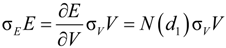where σE is the volatility of equity.
After solving these equations, we can obtain the distance to default and the default probability as:
πMerton = N (−d2) = N (−Merton DD)
where Merton DD is the distance to default and equals d2.
Rather than solving these two nonlinear simultaneous equations to obtain the value and the volatility of the firm’s asset, Bharath and Shumway  directly assign:
V = E + F
and use an approximation to estimate the volatilities:
naive σD = 0.05 + 0.25 ×σE
where naive σD means the volatility of the firm’s debt in the naive model. Hence, the firm’s asset volatility in the naive model, called naive σV, can be represented as:In addition, they replace the risk-free rate in the original model with naive μ, which is the firm’s equity return over the previous year. Finally, the naive distance to default is given as follows:Therefore, we obtain the naive default probability as:
πnaive = N(−Naive DD)

#### 2.2. Merton’s DD Model under Ambiguity

So  derived an adjusted Black–Scholes pricing formula to filter uncertainty from risk in real options analysis by using the robust control technique. If we compare the firm value to the project value in that paper, a similar framework could be applied to solve the problem considered here. In this section, we briefly summarize the theoretical model mentioned in . To save space and concentrate our focus, we omit mentioning proofs here. We recommend that interested readers could refer to  for the more technical parts.
In our model, it is assumed that the risk-free interest rate is r, a constant, and the firm’s value, V, follows a geometric Brownian motion with an expected return μ, dividend yield q and volatility σV:When an investor is under ambiguity and is not confident about probability estimates, a misspecification problem may arise. We use the robust control technique to depict the investor’s ambiguity aversion. The investor would consider an alternative model, Qξ, which is not the same as the reference model, Q. Following Uppal and Wang , we treat the investor as an expected utility-maximizer facing a three-security portfolio (containing the firm, the equity and riskless asset) allocation problem. The Bellman equation is as follows: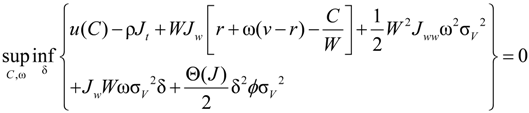where J refers to the expected utility in continuous time. The investor has to choose consumption, C, and asset allocation, ω, to his wealth, W, to maximize his utility. The first term in the second line arises from the change in measure from Q to Qξ, and the term δ adjusts the drift term. The second term in the second line is related to the penalty function, where Θ(J) converts the penalty to units of utility. In addition, we use φ, the penalty parameter, to depict the investor’s confidence about the reference model.
When the investor is under ambiguity, the tendency is to worry about a worst-case scenario. The investor tends to choose alternative models that are more distant from the reference model. Therefore, the robust control approach specifies a lower penalty to more distant perturbations. On the other hand, when the level of the investor’s uncertainty is low, there is a greater likelihood of choosing alternative models that are similar to the reference model. Therefore, the robust control approach specifies a higher penalty to more distant perturbations. The higher is the level of the investor’s ambiguity aversion, the lower is the value of φ. Therefore, the penalty is inversely relative to the investor’s ambiguity aversion, and the reciprocal of φ can be used to describe the investor’s ambiguity aversion.
We assume that the investor has a power utility of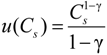and that the value function takes the form:where κ is a constant depending on the parameter of the whole environment. According to Maenhout  and Uppal and Wang , it is assumed that. After taking derivatives of Equation (11) with respect to ω and some computation, we obtain the optimal investment in the firm for the ambiguity-averse investor: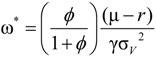The investor’s ambiguity aversion causes a slight downgrade in the risk premium for the firm. Hence, the optimal investment in the firm would be lower than a situation where the data generating process of the firm’s value was known exactly.
By using Ito’s Lemma, we can derive the dynamic process of the investor’s subjective stochastic discount factor under ambiguity for the evaluation of the equity value (that is, the call price):where dBξ is a Brownian motion under the new measure, Qξ. By applying, the dynamic process of the investor’s marginal utility function can be shown to be:By substituting the optimal values of ω*, δ*, and C* we can derive the dynamic process of the investor’s stochastic discount factor under ambiguity as: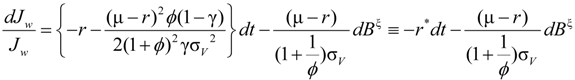where.
We then derive the equity value, E(V,t), under ambiguity. Applying the martingale approach, we have:
EQξ[d(JwE(V,t))] = 0
that is,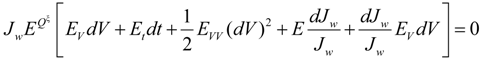By using Equations (16) and (18), this can be rearranged as: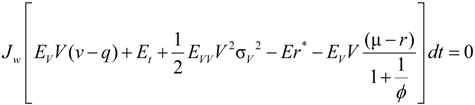The partial differential equation is given as:where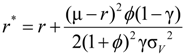and.
Equation (20) can be treated as a typical Black–Scholes partial differential equation with an adjusted risk-free interest rate, r*, and an adjusted dividend yield, q*. Therefore, the equity value under ambiguity can be shown immediately as: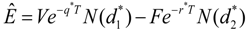where F denotes the face value of the firm’s debt, and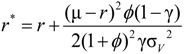,.

#### 2.3. Default Probability under Ambiguity

From Equation (21), the default probability under ambiguity is, whereand. We can see that the default probability of the fixed income, debt, is modified due to investors’ ambiguity aversion. Similarly, the actual probability that a firm will default is computed as π = N(−d2), whereand.
The information of the risk-neutral default probability is critical for pricing newly issued debt or other credit risk derivatives. However, it is not a trivial matter to obtain the risk-neutral default probability when debt is newly issued and only limited market data are available. It is suggested in the literature that the risk-neutral default rate can be implied from the actual default probability by using the mapping: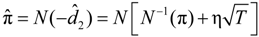, whereis the risk-neutral default probability, and π = N(−d2) is the actual default probability (refer to [28,29,30] for more information of survival analysis). As the default probability under ambiguity is difficult to explore, we applied this approach to imply the default probability under ambiguity from the actual default probability, as follows. The mapping between the two default probabilities is: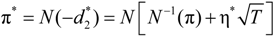By setting, we find that, where φ is the penalty parameter, used to depict the investor’s confidence about the reference model.
As mentioned before, investors’ ambiguity aversion is a subjective parameter, so one cannot easily observe its aggregate value or assign it a reasonable value. Following the literature, we use CCI in our empirical study as a proxy for the penalty parameter, that is:By treating the naive default probability as the actual default probability and using CCI as the value of the penalty parameter, the default probability under ambiguity can be constructed as follows:#### 2.4. Cox Proportional Hazard Model

Hazard models are widely used regression models in a range of survival analyses. Survival analysis focuses on the time period it takes for a specific event to occur. Most importantly, in the literature, such survival models attempt to examine the correlation of survival, or death, with its predictors (called covariates). By definition, the hazard rate is the instantaneous risk of demise at time t. It is also known as the conditional default probability, for example:where T is referred to as a random survival time. In order to examine the relationship between survival and its covariates, the Cox proportional hazard model assigns a linear-form for the log hazard, as follows (see [28,29,30] for further information about survival analysis):
log λi (t) = α (t) + β1xi1 + β2xi2 + … + β k xik
where i is used to identify different individuals, and x’s are the covariates. α(t) = log λ0(t) is referred to as the log-baseline hazard, as it is the value of the log-hazard when all covariates have no effect and are common to all individuals. This model is also useful for an individual-to-individual comparison. The hazard ratio between individual i and j can be defined as:It should be noted that the covariates may vary over time, and the log-hazard with time-dependent covariates is modified as:
log λi (t) = α (t) + β1xi1(t) + β2xi2 (t) + … + β k xik (t)
In order to compare the predictability of πnaive with πCCI, we use a Cox proportional hazard model in our analysis. We want to show that there may be a possible variable other than πnaive in a hazard model that is a statistically significant covariate, so that πnaive is not sufficient for forecasting default. In addition, we have to find evidence to show that πCCI performs better in a hazard model than πnaive. Such findings would support our suggestion that πCCI should be taken into account in forecasting default. As both Campbell, Hilscher and Szilagyi  and Bharath and Shumway  have emphasized that the functional form of the Merton DD model is useful, we also attempt to show that either πnaive or πCCI should retain their statistical significance in a hazard model together with all the variables used to calculate these quantities.

#### 2.5. Credit Default Swap Spread Regression

We also use a market-based default probability variable as a robust test to support the results from the hazard model. A credit default swap (CDS) is a contract to protect bond holders if a default situation occurs. The higher is the probability of default, the higher are the insurance fees payable. Hence, the CDS spread can be considered as a measure of default probability. In this paper, we run regressions of log-CDS spread on log- πnaive or log- πCCI to highlight that πCCI has superior default predictive power.

## 3. Data

The sample is from 2000 to 2011, giving 144 monthly observations, and December, 2007, to 2011 in terms of the CDS spread regression to match the CDS contract. The sample of observed firms was based on an article in CFO Magazine that was published in November, 2003, “Ranking America’s top debt issuers by Moody’s KMV Expected Default Frequency.” We choose 52 firms with complete data as the non-default sample and added 5 firms entering bankruptcy between 2000 and 2011.
Table 1. Summary statistics.
Panel A. Variables in the Merton distance to default (DD) model and hazard models.
Panel A. Variables in the Merton distance to default (DD) model and hazard models.
VariableQuantiles
MeanSDMin0.25Median0.75Max
E59,213.1874,361.400.4513,575.9228,167.4074,009.42519,044.42
F41,562.6157,808.95684.9717,509.2823,839.0038,574.00761,203.00
r (%)2.292.00−0.020.211.724.046.39
CCI86.9428.2225.3061.4092.95106.13144.70
1/σE1.910.440.521.581.922.243.13
naive σv (%)37.0010.0317.9229.8435.3842.4376.44
πnaive (%)6.439.530.000.232.588.2054.21
πCCI (%)6.599.360.000.332.818.5953.56
Panel B. Correlation.
 Corr(πnaive, πCCI) = 0.994
Note: The observation period is from 2000 to 2011. There are 52 non-default firms with 7,488 firm-month observations and 5 default firms with 418 firm-month observations. E and F are the market equity and book debt in millions of dollars, respectively. r is the 3-month risk free rate, and CCI is the Consumer Confidence Index report from the U.S. Conference Board. In addition, 1/σE is the inverse of equity volatility, and r spread is the difference between stock return over the last day of the previous month and the risk-free rate over the last day of the current month. Naive σv is the volatility of firms’ assets calculated by the naive model approach; πnaive is calculated via the naive model, and πCCI isthe default probability with CCI.
We obtained much of the data from the Bloomberg Database, including the 3-month risk-free rate, daily stock prices and shares outstanding. We then calculated the value of firms, the volatility of equity, and stock returns of firms in the USA. Following Bharath and Shumway , we assume that the debts in firms have a one-year horizon. The value of the firms’ debts is obtained from the data of the book value of firms’ total liabilities in Bloomberg. The information of CCI is also available in Bloomberg, which is monthly data, for 7,906 firm-months’ of data, and the data of the firm’s CDS spread were derived from the Datastream database. We adopt a 5-year CDS contract with a base date starting from December, 2007, containing 2,107 firm-months’ of data.
Table 1 shows the descriptive statistics of the variables used in the paper. The information about the variables used in the Merton DD, naive DD and hazard models can be found in Panel A. A simple comparison of πnaive and πCCI is also given in Panel A. Broadly speaking, πCCI has a higher mean and lower standard deviation than does πnaive. From Panel B, we can see that the correlation of πnaive and πCCI is extremely high. Therefore, we can infer that πnaive and πCCI have a high gearing effect.

## 4. Empirical Results

#### 4.1. Hazard Model Results

Table 2 shows the results of the Cox proportional hazard models. Two univariate models and four multivariate models are used. Models 1 and 2 are simple univariate models with dependent variables πnaive and πCCI individually, and two variables are included in Model 3. It is worth noting that πnaive and πCCI are useful for measuring default probabilities, as both are significant in Models 1 and 2, and the coefficients of the two models are negative. In Model 3, although two variables are significant, the sign of πnaive is changed due to the influence of πCCI. Therefore, we can infer that πCCI is a better explanator than πnaive.
Model 4 is a simple reduced form, which contains the same inputs as the naive model and the naive model with uncertainty aversion. Variable CCI is significantly positive, while r spread is not. For this reason, CCI is useful to explain default probability.
Model 5 adds πCCI to Model 4. As πCCI is significant, we infer that the default probability under ambiguity is a useful estimator. In addition, even though all the inputs used to calculate πCCI are contained in the regression, πCCI is still significant. This shows that the functional form of the Merton DD model is quite important. In Model 6, which contains all the variables, both πnaive and πCCI are significant. However, the sign of πnaive is changed owing to the effect of πCCI, under the same conditions as Model 3, suggesting that πCCI is superior to πnaive. As all the variables used to calculate πnaive or πCCI are significant and of the correct sign in Model 6, we confirm robustly that the functional form of the Merton DD model is useful when predicting defaults.
In summary, our findings correspond to those of Campbell, Hilscher and Szilagyi  and Bharath and Shumway , in that the functional form of the Merton DD model is important. Our evidence shows that either πnaive or πCCI is useful in forecasting default. However, πCCI performs slightly better than does πnaive , and this suggests that CCI provides crucial data in default forecasting. Hence, our new default prediction model, which treats investors’ ambiguity aversion as additional information, should be a useful guide for examining default probability.
Table 2. Hazard model estimates.
Table 2. Hazard model estimates.
Dependent Variable: Time to default
VariableModel 1Model 2Model 3Model 4Model 5Model 6
πnaive−0.183 * 0.733 * (0.062) 0.504 *
(0.018)(0.077)
πCCI −21.065 * −97.848 * −22.196 * −76.093 *
(1.981)(7.726)(1.879)(9.071)
CCI 0.021 * 0.015 * 0.013 *
(0.002)(0.002)(0.002)
ln(E) −0.758 * −0.721 * −0.698 *
(0.035)(0.035)(0.035)
ln(F) 0.746 * 0.938 * 0.920 *
(0.048)(0.052)(0.051)
1/σE −2.208 * −2.170 * −2.177 *
(0.168)(0.167)(0.164)
r spread 0.814−3.800 * −3.783 *
(0.342)(0.636)(0.638)
Note: There are 7,906 firm-months in the sample from 2000 to 2011; πnaive is calculated via the naive model; πCCI is the default probability with CCI, and ln(E) and ln(F) are the natural logarithms of market equity and book debt, respectively; 1/σE is the inverse of equity volatility; r spread is the difference between stock return over the last day of the previous month and the 3-month risk free rate over the last day of the current month. Standard errors are shown in parentheses. * Denotes significance at the 1% level.
Panel A. Summary statistics.
Panel A. Summary statistics.
VariableQuantiles
MeanSDMin0.25Median0.75Max
πnaive5.0711.860.000.000.032.7768.63
πCCI5.0211.790.000.000.022.5968.59
Panel B. Regressions.
Panel B. Regressions.
Model 1Model 2Model 3
Constant−1.8494 * (0.0011)−1.6551 * (0.0169)−1.3999 * (0.0776)
log(πnaive)0.1478 * (0.0050) −0.2025 * (0.0599)
log(πCCI) 0.1735 * (0.0058)0.4075 * (0.0695)
Obs.210721072107
R20.29190.29950.3033
Note: The observation period is from December, 2007, to December, 2011. There are 43 firms in the sample for a total of 2,107 firm-month observations. CDS spread is the credit default swap spread in basis points; πnaive is calculated via the naive model; πCCI is the default probability with CCI. Standard errors are shown in parentheses. * Denotes significance at the 1% level.

We also provide evidence from the credit derivatives market to support our empirical findings. The observation period is from December, 2007, to December, 2011, in order to match the five-year CDS contract, which has a base date starting from December, 2007. We have data on 43 firms.
Panel A in Table 3 shows the descriptive statistics of the variables. The CDS spread is in basic points, while πnaive and πCCI are in percentages. We construct three models in this paper, and the details are given in Panel B. In Models 1 and 2, which are simple univariate models, all of the variables are significant, and R2 for in Model 2 is slightly higher than in Model 1. In Model 3, the statistical significance of πnaive is retained, though the sign of the coefficient is changed. However, both the sign and the statistical significance of πCCI are retained. Therefore, πCCI seems to be more suitable for measuring default probability.

## 5. Conclusions

Campbell, Hilscher and Szilagyi  and Bharath and Shumway  provided evidence that the default predictability of the Merton DD model can be attributed to its specific functional form. Bharath and Shumway  constructed a naive default forecasting model. The feature of their naive model is that it retains the functional form of the Merton DD model and computes default probability in a naive way, rather than simultaneously solving two nonlinear equations or implementing a complicated iterative procedure.
Recently, the issue of ambiguity aversion has drawn a lot of attention in the academic field. Although there have been many studies dealing with ambiguity issues in asset prices and equity premia, little is available regarding the influence of ambiguity aversion on forecasting default probability. The purpose of this paper was to construct a default prediction model under ambiguity and to examine the validation of the Merton DD model under ambiguity. To the best of our knowledge, ambiguity aversion has not previously been analyzed in terms of the default prediction issue considered here.
By using CCI in our empirical analysis as an inverse proxy for the level of ambiguity aversion, we constructed a new default forecasting model. Applying the Cox proportional hazard model, this paper showed that the new model was superior to Bharath and Shumway’s naive model. In addition, although the statistical significance of Bharath and Shumway’s naive default probability is retained, its sign is changed due to the effect of our model in the CDS spread regressions. Therefore, our model could serve as a useful reference for modifying the Merton DD model when ambiguity aversion is accommodated.

## Acknowledgments

The authors are very grateful to the Editor-in-Chief, Michael McAleer, and two referees for their very helpful comments and suggestions that improved the quality of our paper.

## Author Contributions

Both authors contributed to all aspects of this work.

## Conflicts of Interest

The authors declare no conflict of interest.

## References

1. R.C. Merton. “On the Pricing of Corporate Debt: The Risk Structure of Interest Rates.” J. Financ. 29 (1974): 449–470. [Google Scholar]
2. J.Y. Campbell, J. Hilscher, and J. Szilagyi. “In Search of Distress Risk.” J. Financ. 63 (2008): 2899–2939. [Google Scholar] [CrossRef]
3. S. Bharath, and T. Shumay. “Forecasting Default with the Merton Distance to Default Model.” Rev. Financ. Stud. 21 (2008): 1339–1369. [Google Scholar] [CrossRef]
4. P. Crosbie, and J. Bohn. “Modeling Default Risk.” 2003. Available online: http://business.illinois.edu/gpennacc/MoodysKMV.pdf (accessed on 20 March 2014).
5. M. Vassalou, and Y. Xing. “Default Risk in Equity Returns.” J. Financ. 59 (2004): 831–868. [Google Scholar] [CrossRef]
6. D. Ellsberg. “Risk, Ambiguity, and Savage Axioms.” J. Econ. 75 (1961): 643–669. [Google Scholar]
7. T.F. Bewley. “Knightian Decision Theory and Econometric Inferences.” J. Econ. Theor. 146 (2011): 1134–1147. [Google Scholar] [CrossRef]
8. J. Dow, and S. R. Werlang. “Uncertainty Aversion, Risk Aversion and the Optimal Choice of Portfolio.” Econometrica 60 (1992): 197–204. [Google Scholar] [CrossRef]
9. L. Epstein, and T. Wang. “Intertemporal Asset Pricing under Knightian Uncertainty.” Econometrica 62 (1994): 283–322. [Google Scholar] [CrossRef]
10. Z. Chen, and L. Epstein. “Ambiguity, Risk and Asset Returns in Continuous Time.” Econometrica 70 (2002): 1403–1443. [Google Scholar] [CrossRef]
11. L. Epstein, and J. Miao. “A Two-Person Dynamic Equilibrium under Ambiguity.” J. Econ. Dyn. Control 27 (2003): 1253–1288. [Google Scholar] [CrossRef]
12. I. Gilboa, and D. Schmeidler. “Maxmin Expected Utility with Non-Unique Prior.” J. Math. Econ. 18 (1989): 141–153. [Google Scholar] [CrossRef]
13. D. Easley, and M. O’Hara. “Microstructure and Ambiguity.” J. Financ. 65 (2010): 1817–1846. [Google Scholar] [CrossRef]
14. T.F. Bewley. “Knightian Decision Theory: Part I.” Decis. Econ. Financ. 25 (2002): 79–110. [Google Scholar] [CrossRef]
15. P. Klibanoff, M. Marinacci, and S. Mukerji. “A Smooth Model of Decision Making under Uncertainty.” Econometrica 73 (2005): 1849–1892. [Google Scholar] [CrossRef]
16. F. Maccheroni, M. Marinacci, and A. Rustinchini. “Ambiguity Aversion, Robustness, and the Variational Representation of Preferences.” Econometrica 74 (2006): 1447–1498. [Google Scholar] [CrossRef]
17. P. Boyle, R. Uppal, T. Wang, and Institute of Finance and Accounting London: London, UK. “Ambiguity Aversion and the Puzzle of Own-Company Stock in Pension Plans.” Working Paper. 2003. [Google Scholar]
18. R. Uppal, and T. Wang. “Model Misspecification and Underdiversification.” J. Financ. 58 (2003): 2465–2486. [Google Scholar] [CrossRef]
19. E. Anderson, L. Hansen, T. Sargent, and Stanford University: Stanford, CA, USA. “Robustness, Detection and the Price of Risk.” Unpublished paper. 2000. [Google Scholar]
20. P. Maenhout. “Robust Portfolio Rules and Asset Pricing.” Rev. Financ. Stud. 17 (2004): 951–983. [Google Scholar] [CrossRef]
21. L. Kogan, T. Wang, and MIT Sloan School of Management: Cambridge, MA, USA. “A Simple Theory of Asset Pricing under Model Uncertainty.” Unpublished Paper. 2002. [Google Scholar]
22. P. Bossaerts, P. Ghirardato, S. Guarneschelli, and W. Zame. “Ambiguity in Asset Markets: Theory and Experiment.” Rev. Financ. Stud. 23 (2010): 1325–1359. [Google Scholar] [CrossRef]
23. L. Epstein, and M. Schneider. “Ambiguity and Asset Markets.” Annu. Rev. Financ. Econ. Annu. Rev. 2 (2010): 315–346. [Google Scholar] [CrossRef]
24. M. Guidolin, and F. Rinaldi. “Ambiguity in Asset Pricing and Portfolio Choice: A Review of the Literature.” Theor. Decis. 74 (2013): 183–217. [Google Scholar] [CrossRef]
25. L. So. “Are Real Options “Real”? Isolating Uncertainty from Risk in Real Options Analysis.” Ann. Financ. Econ., 2014. forthcoming. [Google Scholar]
26. Y. Ait-Sahalia, and M.W. Brandt. “Variable Selection for Portfolio Choice.” J. Financ. 56 (2001): 1297–1351. [Google Scholar] [CrossRef]
27. A. Buraschi, and A. Jiltsov. “Model Uncertainty and Option Markets with Heterogeneous Beliefs.” J. Financ. 61 (2007): 2841–2897. [Google Scholar] [CrossRef]
28. D.R. Cox, and D. Oakes. Analysis of Survival Data. New York, NY, USA: Chapman and Hall, 1984. [Google Scholar]
29. P.D. Allison. Survival Analysis Using the SAS System: A Practical Guide. Cary, NC, USA: SAS Publishing, 1995. [Google Scholar]
30. J. Fox. “Cox Proportional-hazard Regression for Survival Data. Appendix to An R and S-PLUS Companion to Applied Regression.” 2002. Available online: http://cran.r-project.org/doc/contrib/Fox-Companion/appendix-cox-regression.pdf (accessed on 1 February 2014).